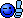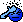# Development Java SE, handling simultaneous Keypresses.

Discussion in 'Software' started by WhiskeyAlpha, 5 Nov 2008.

1. ### WhiskeyAlphaWhat's a Dremel?

Joined:
5 May 2006
Posts:
838
4
Hi guys,

I'm working on a network capable version of the old classic "Pong" for my final major project at Uni (using Netbeans 6.1) and I'm having a few problems already.

Does anybody know of a way of handling simultaneous keyboard input in java? I'm currently using this simple piece of code in a KeyListener:
Code:
```public void keyPressed(KeyEvent e){

if(e.getKeyCode() == e.VK_Q)

if(e.getKeyCode() == e.VK_A)

if(e.getKeyCode() == e.VK_UP)

if(e.getKeyCode() == e.VK_DOWN)

}

public void keyReleased(KeyEvent e){

if(e.getKeyCode() == e.VK_Q)

if(e.getKeyCode() == e.VK_A)

if(e.getKeyCode() == e.VK_UP)

if(e.getKeyCode() == e.VK_DOWN)

} ```
This works reasonably well and I'm able to move both paddles. It does leave me with 2 major problems though:

Firstly (and most importantly) this approach means that only one input can be handled at a time (i.e. both paddles can't be moved simultaneously).

Secondly, there is a noticeable pause between pressing the key that moves a paddle and the actual paddle moving.

Hopefully one of you guys might be able to help a student in distress2. ### theevilelephantMinimodder

Joined:
5 Jan 2006
Posts:
1,334
36
I would say have a set of booleans ie: leftPressed, upPressed, downPressed, right Pressed. use your keyPressed method set a boolean to true (ie if left key was pressed set leftPressed to true), then in your keyReleased method do the opposite. Thats the only way I can think of doing it, i will have a play later and post any working code i getEdit: ok here is a little demo, pressing up and left or up and right at the same time will print out a string
Code:
```import java.awt.event.KeyEvent;
import java.awt.event.KeyListener;
import javax.swing.JFrame;

public class Keys extends JFrame implements KeyListener{
boolean leftP=false;
boolean rightP=false;
boolean upP=false;
boolean downP=false;

public static void main(String[] args){
Keys k = new Keys();
}

Keys(){
this.setDefaultCloseOperation(EXIT_ON_CLOSE);
this.setVisible(true);
while(true){
if((leftP)&&(upP)){
System.out.println("Left and Up");
leftP=false; upP=false;
}
else if((rightP)&&(upP)){
System.out.println("Right and Up");
rightP=false; upP=false;
}
}
}

public void keyPressed(KeyEvent e) {
if(e.getKeyCode() == e.VK_LEFT){
leftP=true;
}

if(e.getKeyCode() == e.VK_RIGHT){
rightP=true;
}

if(e.getKeyCode() == e.VK_UP){
upP=true;
}

if(e.getKeyCode() == e.VK_DOWN){
downP=true;
}

if(e.getKeyCode() == e.VK_Q){
System.exit(0);
}
}

public void keyReleased(KeyEvent e) {
if(e.getKeyCode() == e.VK_LEFT){
leftP=false;
}

if(e.getKeyCode() == e.VK_RIGHT){
rightP=false;
}

if(e.getKeyCode() == e.VK_UP){
upP=false;
}

if(e.getKeyCode() == e.VK_DOWN){
downP=false;
}

}

public void keyTyped(KeyEvent arg0) {
// TODO Auto-generated method stub

}

}
```

Last edited: 5 Nov 2008
3. ### WhiskeyAlphaWhat's a Dremel?

Joined:
5 May 2006
Posts:
838
4
Thanks theevilelephantI've actually developed something very similar to what you suggest and have a different set of problems nowCode:
```public void keyPressed(KeyEvent e){

if(e.getKeyCode() == e.VK_Q){
else if(e.getKeyCode() == e.VK_A){
else if(e.getKeyCode() == e.VK_UP){
else if(e.getKeyCode() == e.VK_DOWN){

multipleKeyCheck();
singleKeyCheck();

}

public void singleKeyCheck(){
}

public void multipleKeyCheck(){
}

public void keyReleased(KeyEvent e){

if(e.getKeyCode() == e.VK_Q)
if(e.getKeyCode() == e.VK_A)
if(e.getKeyCode() == e.VK_UP)
if(e.getKeyCode() == e.VK_DOWN)
} ```
New problems:

1) when both paddles are moving simultaneously, the left paddle appears to move about twice as fast - semi-fixed - this is because the singleKeyCheck and multipleKeyCheck are both being processed and the left paddle gets moved first in singleKeyCheck, I believe.
2) occasionally the right paddle won't move down - fixed
3) various other weird behaviour - i.e. if both paddles are moving and then you lift off of one key, both paddles stop. once you reapply the key that you lifted off of, both panels move normally.
4) the "delay" that is present at the first press (when holding the key) when using a keylistener for this job still troubles me. I wonder if there is a method to reduce it?

probs just need to have a good look through my code and work out what's what

Last edited: 5 Nov 2008
4. ### theevilelephantMinimodder

Joined:
5 Jan 2006
Posts:
1,334
36
3) im guessing this is because you call the multipleKeyCheck in the key press method
4) i have no idea, my dem doesnt seem to experience a delay

Here is a slightly different version of my code. If you hold left and up "Left and Up" will be printed untill you let go, if you let go of either Left or Up then either "Left" or "up" will be printed untill you either let go or press another key. Im fairly sure this is what you are after? Compile it an have a play, see what you think
Code:
```import java.awt.event.KeyEvent;
import java.awt.event.KeyListener;
import javax.swing.JFrame;

public class Keys extends JFrame implements KeyListener{
boolean leftP=false;
boolean rightP=false;
boolean upP=false;
boolean downP=false;

public static void main(String[] args){
Keys k = new Keys();
}

Keys(){
this.setDefaultCloseOperation(EXIT_ON_CLOSE);
this.setVisible(true);
while(true){
if((leftP)&&(upP)){
System.out.println("Left and Up");
//leftP=false; upP=false;
}
else if((rightP)&&(upP)){
System.out.println("Right and Up");
//rightP=false; upP=false;
}
else if(leftP){
System.out.println("left");
}
else if(upP){
System.out.println("Up");
}
}
}

public void keyPressed(KeyEvent e) {
if(e.getKeyCode() == e.VK_LEFT){
leftP=true;
}

if(e.getKeyCode() == e.VK_RIGHT){
rightP=true;
}

if(e.getKeyCode() == e.VK_UP){
upP=true;
}

if(e.getKeyCode() == e.VK_DOWN){
downP=true;
}

if(e.getKeyCode() == e.VK_Q){
System.exit(0);
}
}

public void keyReleased(KeyEvent e) {
if(e.getKeyCode() == e.VK_LEFT){
leftP=false;
}

if(e.getKeyCode() == e.VK_RIGHT){
rightP=false;
}

if(e.getKeyCode() == e.VK_UP){
upP=false;
}

if(e.getKeyCode() == e.VK_DOWN){
downP=false;
}

}

public void keyTyped(KeyEvent arg0) {}

}
```

5. ### dfhaiiinternets

Joined:
24 Mar 2002
Posts:
520
0
Code:
```import java.awt.event.KeyAdapter;
import java.awt.event.KeyEvent;
import javax.swing.JFrame;

public class Keys extends JFrame {
volatile boolean mLeftPressed;
volatile boolean mRightPressed;
volatile boolean mUpPressed;
volatile boolean mDownPressed;

public static void main(String[] args){
Keys k = new Keys();
}

public Keys(){
super("KeyTest");
this.setDefaultCloseOperation(EXIT_ON_CLOSE);
this.setVisible(true);
@Override
public void keyPressed(KeyEvent e) {
switch(e.getKeyCode()){
case KeyEvent.VK_LEFT:
mLeftPressed = true;
break;
case KeyEvent.VK_RIGHT:
mRightPressed = true;
break;
case KeyEvent.VK_UP:
mUpPressed = true;
break;
case KeyEvent.VK_DOWN:
mDownPressed = true;
break;
case KeyEvent.VK_Q:
System.exit(0);
break;
}
}

@Override
public void keyReleased(KeyEvent e) {
switch(e.getKeyCode()){
case KeyEvent.VK_LEFT:
mLeftPressed = false;
break;
case KeyEvent.VK_RIGHT:
mRightPressed = false;
break;
case KeyEvent.VK_UP:
mUpPressed = false;
break;
case KeyEvent.VK_DOWN:
mDownPressed = false;
break;
case KeyEvent.VK_Q:
System.exit(0);
break;
}
}
});

public void run(){
while(true){
//check for your buttons being pressed here
if(mLeftPressed)
System.out.println("LEFT");
if(mRightPressed)
System.out.println("RIGHT");
if(mUpPressed)
System.out.println("UP");
if(mDownPressed)
System.out.println("DOWN");
try{
}catch(InterruptedException e){}
}
}
};
KeyPressedMonitor.start();
}
}
```
Something like that might work for you? I suspect you're probably having problems with your gui due to not ensuring updates to swing components are happening on the EDT, and by using the EDT to do heavy work killing it momentarily.

I've not tried even building that code, but I'm fairly sure it will and it'll supply you with a set of bools so you know which buttons are down at any one time.

Last edited: 27 Nov 2008

Joined:
30 Apr 2002
Posts:
1,669